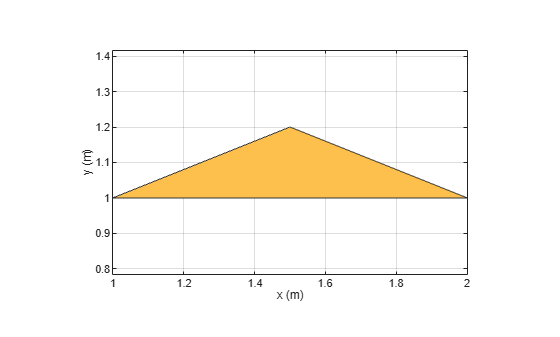# antenna.Polygon

Create polygon on X-Y plane

## Description

Use the `antenna.Polygon` object to create a polygonal board shape centered at the origin and on the X-Y plane. You can use `antenna.Polygon` to create single-layer or multi-layered antennas using `pcbStack`.

## Creation

### Syntax

``polygon = antenna.Polygon``
``polygon = antenna.Polygon(Name=Value)``

### Description

````polygon = antenna.Polygon` creates a polygonal board shape centered at the origin and on the X-Y plane.```

example

````polygon = antenna.Polygon(Name=Value)` sets properties using one or more name-value arguments. `Name` is the property name and `Value` is the corresponding value. You can specify several name-value arguments in any order as `Name1`= `Value1`, `...`, `NameN`=`ValueN`. Properties not specified retain their default values.```

## Properties

expand all

Name of the polygon board shape, specified as a string or a character vector.

Example: `"Polygon1"`

Data Types: `char` | `string`

Cartesian coordinates of polygon vertices, specified as a N-by-3 matrix with each element measured in meters, N being the number of points.

Example: `[-1 0 0; -0.5 0.2 0; 0 0 0]`

Data Types: `double`

## Object Functions

 `add` Boolean unite operation on two shapes `subtract` Boolean subtraction operation on two shapes `intersect` Boolean intersection operation on two shapes `plus` Shape1 + Shape2 `minus` Shape1 - Shape2 `and` Shape1 & Shape2 `area` Calculate area of shape in square meters `show` Display antenna, array structures or shapes `plot` Plot boundary of shape `mesh` Mesh properties of metal, dielectric antenna, or array structure `rotate` Rotate shape about axis and angle `rotateX` Rotate shape about x-axis and angle `rotateY` Rotate shape about y-axis and angle `rotateZ` Rotate shape about z-axis and angle `translate` Move shape to new location `scale` Change the size of the shape by a fixed amount `mirrorX` Mirror shape along X-axis `mirrorY` Mirror shape along Y-axis `removeHoles` Remove holes from shape `removeSlivers` Remove sliver outliers from boundary of shape

## Examples

collapse all

Create a polygon using `antenna.Polygon` with vertices at `[-1 0 0; -0.5 0.2 0; 0 0 0]` and display it.

`p = antenna.Polygon(Vertices=[-1 0 0; -0.5 0.2 0; 0 0 0])`
```p = Polygon with properties: Name: 'mypolygon' Vertices: [3x3 double] ```
```show(p) axis equal```Mesh the polygon and display the meshed shape.

`mesh(p,0.2)`Move the polygon to a new location on the X-Y plane.

```translate(p,[2,1,0]) axis equal```## Version History

Introduced in R2017a